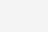# 实验三：掌握Pandas基本操作

## 【实验内容】

1、安装pandas。

2、掌握中pandas中Series和Dataframe对象的创建以及使用。

3、使用pandas读取csv文件，并做简单分析。

## 【实验目的】

1、掌握pandas对数据的基本处理方法。

2、掌握pandas对csv文件的处理操作。

## 【实验步骤】

### 步骤1：安装pandas库。

```#方法一，直接在终端输入。
pip install pandas
#方法二，在jupyter notebook中输入。
!pip install pandas```

### 步骤2：掌握Series（一维数据）的操作。

Series 是一种类似于一维数组的对象，它由一组数据（各种Numpy数据类型）以及一组与之相关的数据标签（即索引）组成。

```#导入所需库。
import numpy as np
import pandas as pd```
```s1 = pd.Series(np.arange(1,4))  #创建series，左边一列为索引，默认从0开始。
s1```

1    2

2    3

dtype: int32

```#以数组的形式创建，并指定其索引。
s2 = pd.Series([1,2,3],index=['A','B','C'])
s2```

B    2

C    3

dtype: int64

```#按照默认索引和指定索引进行查找其对应元素。
print(s2)
print(s2['B'])```

2

```#设置series的参数名称。
dict1 = {'a':18, 'b':22, 'c':24}
pd.Series(dict1, name='age')```

b    22

c    24

Name: age, dtype: int64

### 步骤3：掌握Dataframe（二维数据）的操作。

DataFrame 是一个表格型的数据结构，它含有一组有序的列，每列可以是不同的值类型(数值、字符串、布尔型值)。DataFrame 既有行索引也有列索引，它可以被看做由 Series 组成的字典（共同用一个索引）。

```#创建DataFrame。
s1 = pd.Series(['张三', 18, '男', '武汉', '打球'])
s2 = pd.Series(['李四', 22, '女', '上海', '跑步'])
s3 = pd.Series(['王五', 24, '男', '杭州', '学习'])
s4 = pd.Series(['老六', 13, '女', '天津', '游泳'])
s5 = pd.Series(['无名', 23, '男', '深圳', '写代码'])
df1 = pd.DataFrame([s1,s2,s3,s4,s5])
df1``````#修改索引名和列名。
df1.index= ['A', 'B', 'C', 'D', 'E']
df1.columns = ['name', 'age', 'sex', 'address', 'hobby']
df1``````#通过字典的形式创建。
df2 = pd.DataFrame({
'name' : ['张三', '李四', '王五', '老六', '无名'],
'age' : [18, 22, 24, 13, 23],
'sex' : ['男', '女', '男', '女', '男'],
'address' : ['武汉', '上海', '杭州', '天津', '深圳'],
'hobby' : ['打球', '跑步', '学习', '游泳', '写代码']
}, index=['A', 'B', 'C', 'D', 'E'])
df2``````#删除指定列。
df2``````#通过切片查看指定位置元素。
df2.iloc[2:5]````df2.iloc[:,-1]`

B     跑步

C     学习

D     游泳

E    写代码

Name: hobby, dtype: object

### 步骤4：掌握pandas对csv文件的操作。

CSV（Comma-Separated Values，逗号分隔值，有时也称为字符分隔值，因为分隔字符也可以不是逗号），其文件以纯文本形式存储表格数据（数字和文本）。

CSV 是一种通用的、相对简单的文件格式，被用户、商业和科学广泛应用。

```# 读取csv，此数据为波士顿房价数据。
df``````#查看前5行数据。```#查看数据信息。
df.info()``````#查看各个字段的数量、均值、标准差、最小值、四分位数、最大值等。
df.describe()``````#筛选出CRIM大于0.5并且ZN大于0的字段。
df = df[(df['CRIM']>0.5)&(df['ZN']>0)]
df``````#导出csv文件并重命名为df。
df.to_csv('df',index=0)```### 步骤5：掌握对缺失值的处理方法。

isnull()  为空  没有数据显示True,有数据显示False。

notnull() 不为空 有数据显示True。

fillna()  填充缺失值。

dropna()  去除缺失值。

```#创建含有缺失值的数据。
#创建含有缺失值的数据。
import numpy as np
df = pd.DataFrame({
'name' : ['tom', 'poppy', 'alex'],
'age' : [23, np.NaN, 30],
'score' : [np.NaN, 18, np.NaN],
'hobby' : ['吃饭', np.NaN, '写代码']
})
df``````#isnull判断是否为缺失值，是则返回True，否则返回False。
df.isnull()``````#删除含有缺失值的列。
df.dropna(axis=1)``````#填充指定值。
df.fillna(0)``````#用前一个值或后一个值来填充。
print(df.fillna(method='ffill'))
print(df.fillna(method='bfill'))```### 联系我们

##### 027-87870986